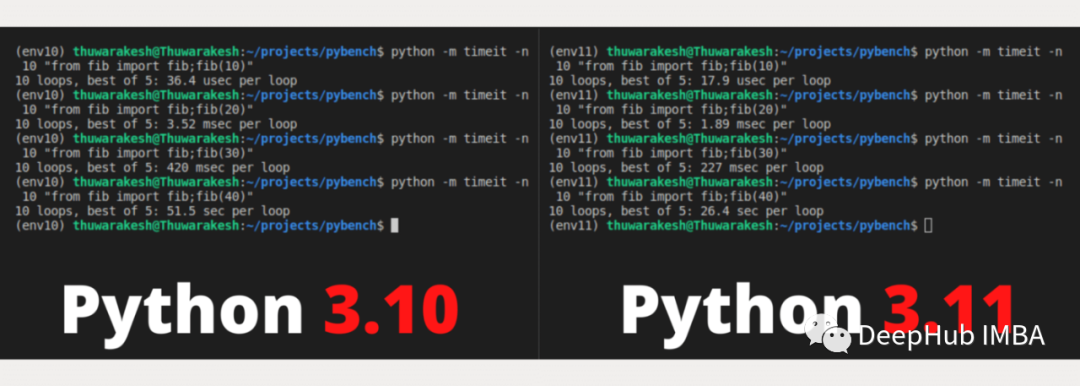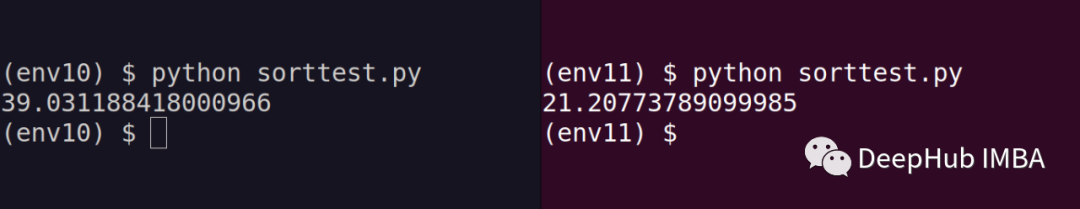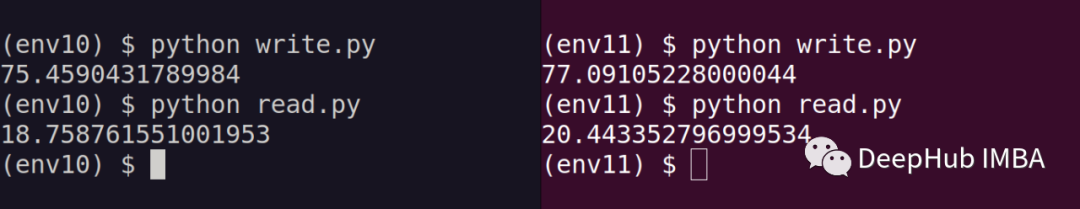0

# Python 3.11比3.10 快60%：使用冒泡排序和递归函数对比测试

Python 3.11 pre-release已经发布。更新日志中提到：

Python 3.11 is up to 10–60% faster than Python 3.10. On average, we measured a 1.25x speedup on the standard benchmark suite. See Faster CPython for details. — Python 3.11 Changelog.

Python 在生产系统上的速度一直是被新手对比和吐槽。，因为真的并不块，为了解决性能问题，我们总是需要使用 Cython 或 Tuplex 转换关键代码。

Python 3.11中特意强了这个优化，我们可以实际验证下到底有没有官方说的平均1.25倍的提升呢？

## 安装Python 3.11 pre-release

windows的话可以在官方下载安装文件，ubuntu可以用apt命令进行安装

``````sudo apt install Python3.11
``````

``````\$ virtualenv env10 --python=3.10
\$ virtualenv env11 --python=3.11

# To activate v11 you can run,
\$ source env11/bin/activate
``````

## Python 3.11 与 Python 3.10 相比有多快？

``````def fib(n: int) -> int:
return n if n < 2 else fib(n - 1) + fib(n - 2)
``````

``````# To generate the (n)th Fibonacci number
python -m timeit -n 10 "from fib import fib;fib(n)"
``````Python 3.11 在每次运行中都优于 Python 3.10。执行时间大约是 3.11 版本的一半。

## 冒泡排序

``````import random
from timeit import timeit
from typing import List

def bubble_sort(items: List[int]) -> List[int]:
n = len(items)

for i in range(n - 1):

for j in range(0, n - i - 1):

if items[j] > items[j + 1]:
items[j], items[j + 1] = items[j + 1], items[j]

numbers = [random.randint(1, 10000) for i in range(1000000)]

print(timeit(lambda:bubble_sort(numbers),number=5))
``````Python 3.11 只用了 21 秒来排序，而 3.10 对应的用时 39 秒。

## I/O 操作是否存在性能差异？

``````from timeit import timeit

statement = """
for i in range(100000):
with open(f"./data/a{i}.txt", "w") as f:
f.write('a')
"""

print(timeit(statement, number=10))
``````

``````from glob import glob
from timeit import timeit

file_paths = glob("./data/*.txt")

statement = f"""
for path in {file_paths}:
with open(path, "r") as f:
"""

print(timeit(statement, number=10))
``````## 总结

Python 3.11 仍然是一个预发布版本。3但它似乎是 Python 历史上一个了不起的版本。它比之前的版本快了 60%，这个判断还是没毛病的，我们上面的一些实验也证明了 Python 3.11 确实更快。

### “Python 3.11比3.10 快60%：使用冒泡排序和递归函数对比测试”的评论:

##### 关于作者##### Deephub